# Grammar Ambiguity | Check Ambiguous Grammar

## Grammar Ambiguity-

Before you go through this article, make sure that you have gone through the previous article on Ambiguous Grammar.

• There exists no algorithm to check whether any given grammar is ambiguous or not.
• This general decision problem is undecidable-

“Whether a grammar is ambiguous or not?”

• This is because it can be shown that this problem is equivalent to Post Correspondence Problem.

## General Approach To Check Grammar Ambiguity-

To check whether a given grammar is ambiguous or not, we follow the following steps-

### Step-01:

We try finding a string from the Language of Grammar such that for the string there exists more than one-

• parse tree
• or derivation tree
• or syntax tree
• or leftmost derivation
• or rightmost derivation

### Step-02:

If there exists at least one such string, then the grammar is ambiguous otherwise unambiguous.

## Problem-01:

Check whether the given grammar is ambiguous or not-

S → SS

S → a

S → b

## Solution-

Let us consider a string w generated by the given grammar-

w = abba

Now, let us draw parse trees for this string w.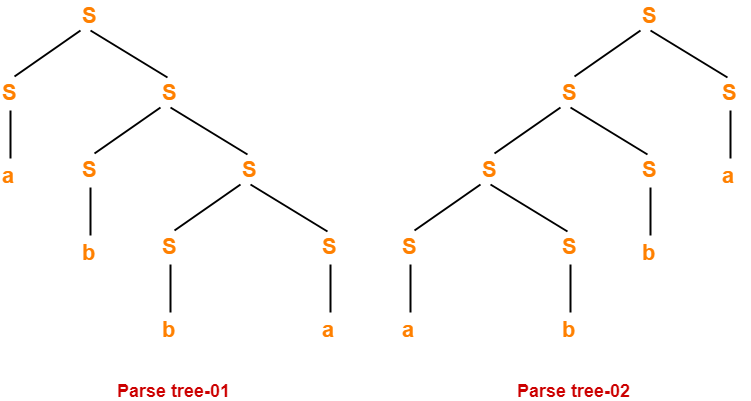Since two different parse trees exist for string w, therefore the given grammar is ambiguous.

## Problem-02:

Check whether the given grammar is ambiguous or not-

S → A / B

A → aAb / ab

B → abB / ∈

## Solution-

Let us consider a string w generated by the given grammar-

w = ab

Now, let us draw parse trees for this string w.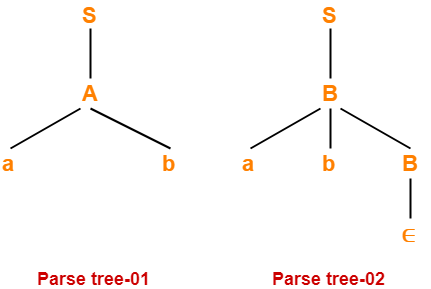Since two different parse trees exist for string w, therefore the given grammar is ambiguous.

## Problem-03:

Check whether the given grammar is ambiguous or not-

S → AB / C

A → aAb / ab

B → cBd / cd

C → aCd / aDd

D → bDc / bc

## Solution-

Let us consider a string w generated by the given grammar-

w = aabbccdd

Now, let us draw parse trees for this string w.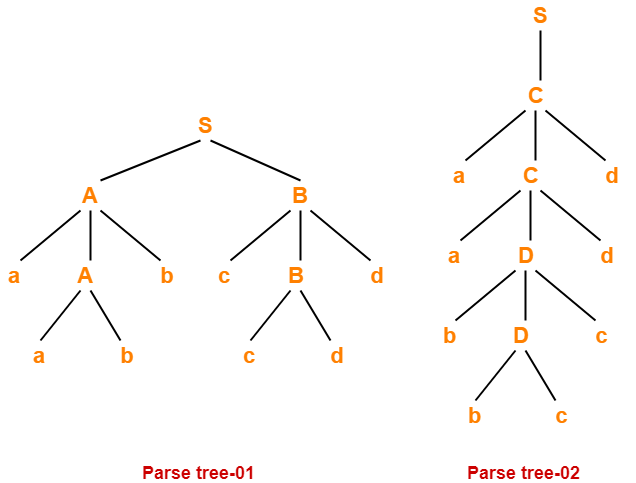Since two different parse trees exist for string w, therefore the given grammar is ambiguous.

## Problem-04:

Check whether the given grammar is ambiguous or not-

S → AB / aaB

A → a / Aa

B → b

## Solution-

Let us consider a string w generated by the given grammar-

w = aab

Now, let us draw parse trees for this string w.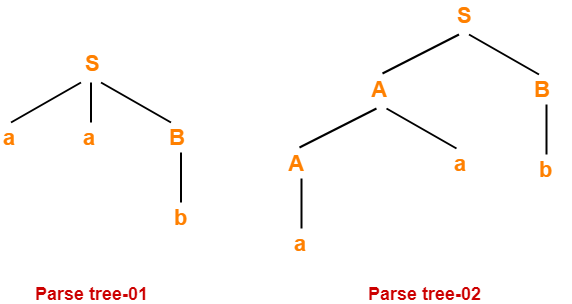Since two different parse trees exist for string w, therefore the given grammar is ambiguous.

## Problem-05:

Check whether the given grammar is ambiguous or not-

S → a / abSb / aAb

A → bS / aAAb

## Solution-

Let us consider a string w generated by the given grammar-

w = abababb

Now, let us draw parse trees for this string w.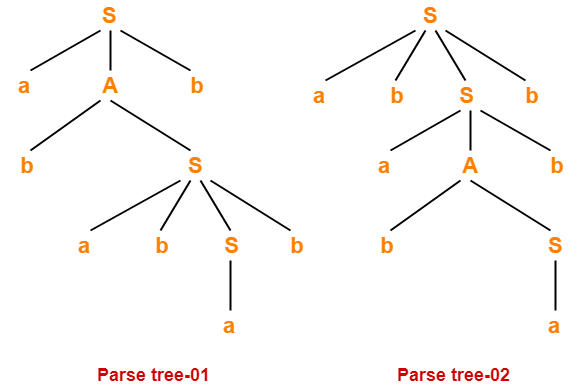Since two different parse trees exist for string w, therefore the given grammar is ambiguous.

## Problem-06:

Check whether the given grammar is ambiguous or not-

E → E + T / T

T → T x F / F

F → id

## Solution-

• There exists no string belonging to the language of grammar which has more than one parse tree.
• Since a unique parse tree exists for all the strings, therefore the given grammar is unambiguous.

## Problem-07:

Check whether the given grammar is ambiguous or not-

S → aSbS / bSaS / ∈

## Solution-

Let us consider a string w generated by the given grammar-

w = abab

Now, let us draw parse trees for this string w.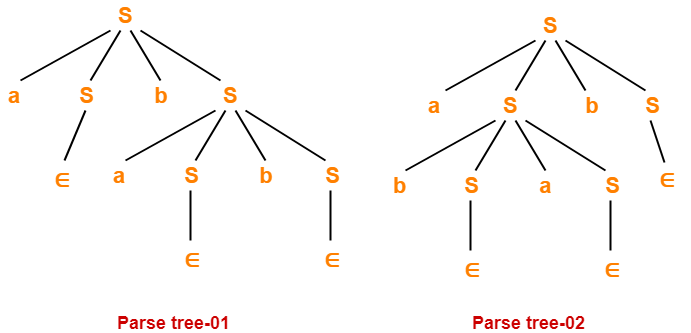Since two different parse trees exist for string w, therefore the given grammar is ambiguous.

## Problem-08:

Check whether the given grammar is ambiguous or not-

R → R + R / R . R / R* / a / b

## Solution-

Let us consider a string w generated by the given grammar-

w = ab + a

Now, let us draw parse trees for this string w.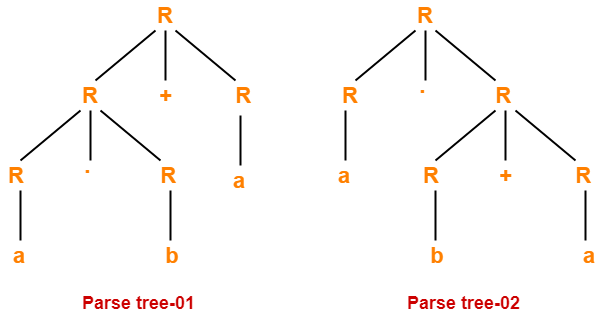Since two different parse trees exist for string w, therefore the given grammar is ambiguous.

To gain better understanding about Grammar Ambiguity,

Watch this Video Lecture

Next Article- Converting Ambiguous into Unambiguous Grammar

Get more notes and other study material of Theory of Automata and Computation.

Watch video lectures by visiting our YouTube channel LearnVidFun.

SummaryArticle Name
Grammar Ambiguity | Check Ambiguous Grammar
Description
Check Whether Grammar is Ambiguous or Not- To check grammar ambiguity, we try to find one string for which there exists more than one parse tree. There exists no algorithm to check whether grammar is ambiguous or not.
Author
Publisher Name
Gate Vidyalay
Publisher Logo